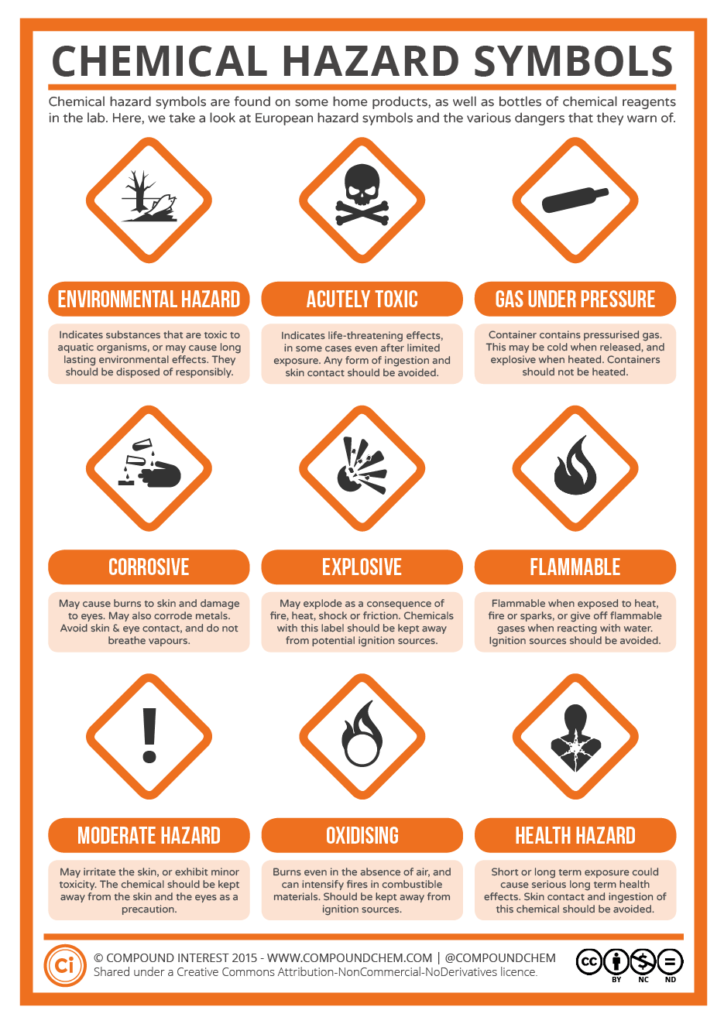## LEARNING OBJECTIVES

• Describe proper laboratory attire and behavior.
• Identify laboratory equipment and define its purpose.
• Argue a scientific claim through presentation of evidence and reasoning.
• Identify the independent and dependent variable in an experiment.
• Identify what a chemical property is and be able to give some examples.
• Identify what a physical property is and be able to give some examples.
• Describe how a physical change occurs and how it is different from a chemical change.
• Describe how a chemical change occurs and know the indicators of a chemical reaction.
• Explain the differences between a substance and a mixture.
• Compare and contrast homogeneous and heterogeneous matter.
• Identify the difference between the particle movement in a solid, liquid, and a gas.
• Name each phase change and specifically describe which states of matter are involved.
• Determine which separation technique would best isolate the components in a mixture.
• Know the metric base units for time, temperature, length, mass, and volume.
• Identify and use SI prefixes (mega, kilo, deci, centi, milli, micro, nano).
• Describe the difference between accuracy and precision.
• Read measurement devices in the laboratory environment and estimate to the correct digit.
• Determine which digits in a measurement are significant.
• Set up a line graph, placing the independent and dependent variables on the appropriate axis.
• Convert small decimals and large whole numbers to scientific notation and vice versa.
• Round a calculated value to the appropriate number of significant figures.
• Use the formula for percent error including identifying the actual and experimental values.
• Label all answers with the proper unit.
• Solve the density equation for density, mass, and volume.

## VIDEO

Metric Conversions

Density Calculations

Significant Figures

Physical & Chemical Properties & Changes

Operations with Scientific Notation

Scientific Notation

Classifying Matter

## SIMULATIONS

Explore States of Matter at PhET

Explore Density, Mass & Volume at PhET

## WEBSITES

NFPA Diamond

Practice Identifying Variables

Printable Ruler